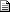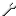# Maths - Matrix algebra

 By: Nobody/Anonymous - nobodyRight Hand to Left Hand Matrix   2005-07-19 15:35 Greetings!    I have run into some conversion problems. I thought that a 4x4 matrix like that:  R R R 0  R R R 0  R R R 0  X Y Z 0    can be multiplied by:  1 0 0 0  0 0 1 0  0 1 0 0  0 0 0 1    Which "should" produce a LEFT handed matrix. It sometimes works and sometimes it does not work. I do not know why it happens.    Is this method false? Does anyone have a suggestion how to fix that issue? Some say just flip z by z *= -1 and the same for rotations? The big "R" in my matrx is a rotation matrix.    Thank you in advance!

 By: Martin Baker - martinbakerRE: Right Hand to Left Hand Matrix   2005-07-19 21:11 A right handed coordinate system can be converted to a left handed coordination system (or via versa) by either:  Inverting any one coordinate basis (such as replacing z with -z)  or swapping any two coordinate basis (such as x and y).    However I'm not sure you can change a matrix which does some function in a right hand coordinate system to make it do a similar function in a left hand system in the way you suggest.    For instance the top row in the matrix is associated with 'x' dimension and the left column is also associated with 'x' dimension so we need to swap both rows and columns and I suspect other things may also need to be changed. So I think the change to the matrix may be quite complex.    Martin
 By: Nobody/Anonymous - nobodyRE: Right Hand to Left Hand Matrix   2005-07-20 11:13 Hm I see your point, but what to do with rotations? I have yaw, pitch, roll. Shall I invert the roll to -roll to make it right? I need matrix that contains as well rotations as translations.    Thank you for your reply!
 By: Martin Baker - martinbakerRE: Right Hand to Left Hand Matrix   2005-07-20 16:59 If you want convert Euler angle to a matrix using left hand coodrinate system you should be able to derive the matrix using the standard you want to use in a similar way that the right hand matrix is derived on this page:    https://www.euclideanspace.com/maths/geometry/rotations/conversions/eulerToMatrix/    All this involves is working out the matrix for yaw, pitch and roll individually using left hand coordinate. Then multiply these individual matrices in the order appropriate for the type of euler angles you want to use (yaw, pitch, roll).    Martin
 By: Nobody/Anonymous - nobodyRE: Right Hand to Left Hand Matrix   2006-01-15 00:37 If you want to convert a matrix that does a function in right-handed space to do the same function in left-handed space, you need to invert both z-column and z-row (which implies that the  element is unchanged).    Look up the "similarity transform"   converted_function = matrix_into_space * function * matrix_from_space
 By: Martin Baker - martinbakerRE: Right Hand to Left Hand Matrix   2006-01-15 16:13 > If you want to convert a matrix that does a function in right-handed space to do the same function in  > left-handed space, you need to invert both z-column and z-row (which implies that the  element  > is unchanged).     If we are converting from right->left by inverting the z coordinate, then I think that in addition to inverting both z-column and z-row I suspect we also need to invert any z-terms in the body of the matrix. Would you agree with this? In this case, since we are talking about euler angle to matrix, then I suggest this means we also invert attitude (rotate about z)?    Martin
 By: Nobody/Anonymous - nobodyRE: Right Hand to Left Hand Matrix   2006-01-15 17:43 Just write down the similarity transform and do the math. For left/right conversion, both the matrix that converts into the custom space and it's inverse are:    1 0 0 0  0 1 0 0  0 0 -1 0  0 0 0 1